## How to sum values based on text criteria in Excel?

In Excel, have you ever tried to sum values based on another column of text criteria? For example, I have a range of data in worksheet as following screenshot shown, now, I want to add up all the numbers in column B corresponding with the text values in column A that meet a certain criterion, such as sum the numbers if the cells in column A contains KTE.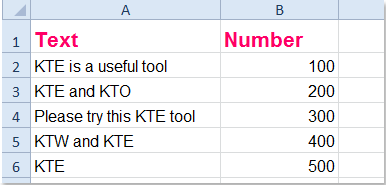Sum the values based on another column if contains certain text Sum the values based on another column if starts with certain text Sum the values based on another column if ends with certain text Sum the values based on another column if only is certain text

####Sum the values based on another column if contains certain text

Let’s take the above data for example, to add all the values together which contains the text “KTE” in column A, the following formula may help you:

Please enter this formula =SUMIF(A2:A6,"*KTE*",B2:B6) into a blank cell, and press Enter key, then all the numbers in column B where the corresponding cell in column A contains text “KTE” will add up. See screenshots: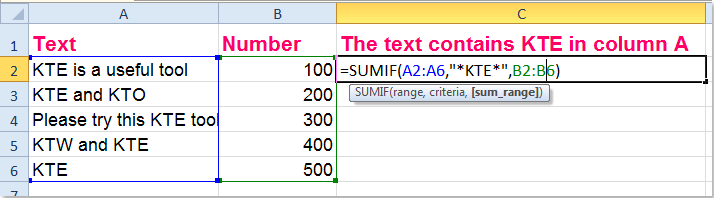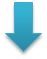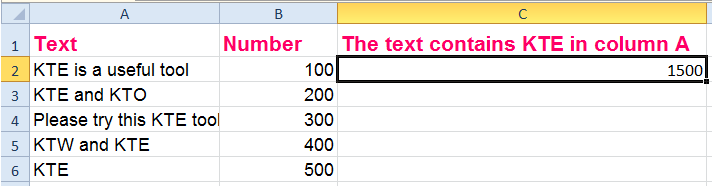Tip: In the above formula, A2:A6 is the data range which you add the values based on, *KTE* stands for the criteria you need, and B2:B6 is the range you want to sum.

####Sum the values based on another column if starts with certain text

If you just want to sum the cell values in column B where the corresponding cell in column A which text starts with “KTE”, you can apply this formula: =SUMIF(A2:A6,"KTE*",B2:B6), see screenshots: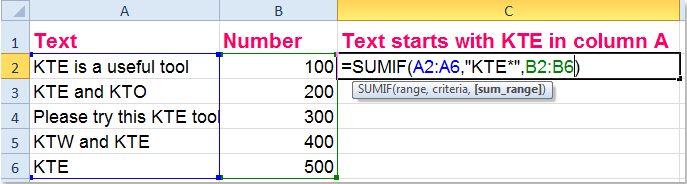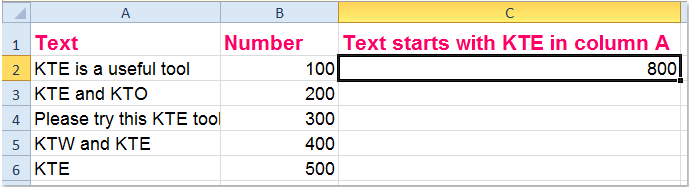Tip: In the above formula, A2:A6 is the data range which you add the values based on, KTE* stands for the criterion you need, and B2:B6 is the range you want to sum.

####Sum the values based on another column if ends with certain text

To add up all the values in column B where the corresponding cell in column A which text ends with “KTE”, this formula can do you a favor: =SUMIF(A2:A6,"*KTE",B2:B6), (A2:A6 is the data range which you add the values based on, KTE* stands for the criterion you need, and B2:B6 is the range you want to sum)see screenshots: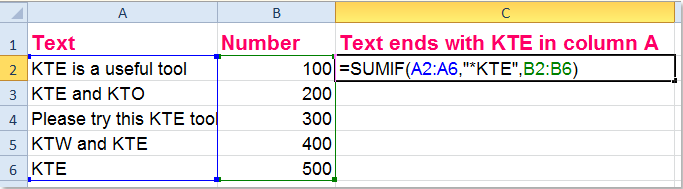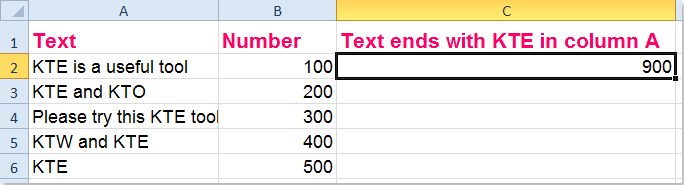####Sum the values based on another column if only is certain text

If you just want to sum the values in column B which corresponding cell content only is “KTE” of column A, please use this formula: =SUMIF(A2:A6,"KTE",B2:B6), (A2:A6 is the data range which you add the values based on, KTE stands for the criterion you need, and B2:B6 is the range you want to sum) and then only the text is “KTE” in column A which relative number in column B will add up, see screenshots: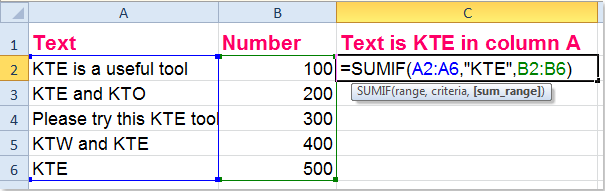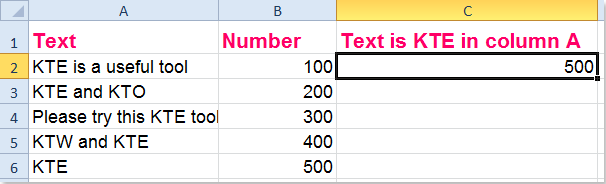Advanced Combine Rows:(Combine duplicate rows and sum / average corresponding values): 1. Specify the key column that you want to combine other column based on; 2. Choose one calculation for your combined data.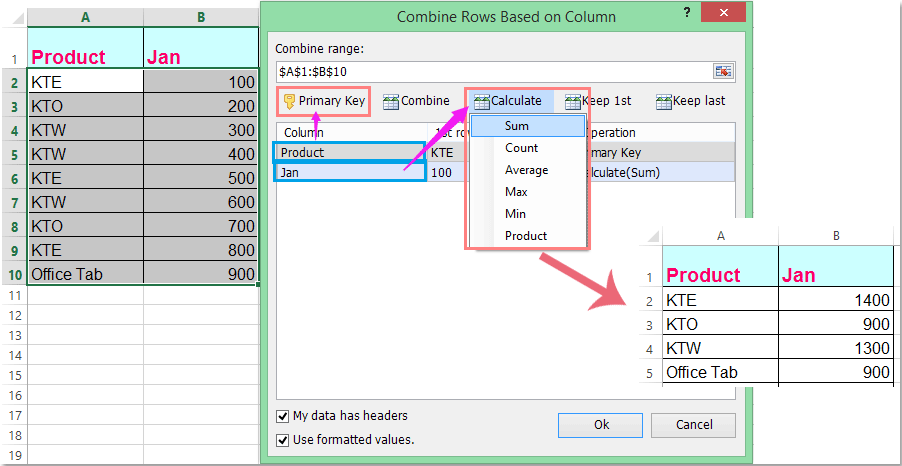Kutools for Excel: with more than 200 handy Excel add-ins, free to try with no limitation in 60 days.

Related articles:

How to sum every n rows down in Excel?

How to sum cells with text and numbers in Excel?

### Best Office Productivity Tools

 Popular Features: Find, Highlight or Identify Duplicates   |  Delete Blank Rows   |  Combine Columns or Cells without Losing Data   |   Round without Formula ... Super Lookup: Multiple Criteria VLookup  |   Multiple Value VLookup  |   VLookup Across Multiple Sheets   |   Fuzzy Lookup .... Advanced Drop-down List: Quickly Create Drop Down List   |  Dependent Drop Down List   |  Multi-select Drop Down List .... Column Manager: Add a Specific Number of Columns  |  Move Columns  |  Toggle Visibility Status of Hidden Columns  |  Compare Ranges & Columns ... Featured Features: Grid Focus   |  Design View   |   Big Formula Bar   |  Workbook & Sheet Manager   |  Resource Library (Auto Text)   |  Date Picker   |  Combine Worksheets   |  Encrypt/Decrypt Cells   |  Send Emails by List   |  Super Filter   |   Special Filter (filter bold/italic/strikethrough...) ... Top 15 Toolsets:  12 Text Tools (Add Text, Remove Characters, ...)   |   50+ Chart Types (Gantt Chart, ...)   |   40+ Practical Formulas (Calculate age based on birthday, ...)   |   19 Insertion Tools (Insert QR Code, Insert Picture from Path, ...)   |   12 Conversion Tools (Numbers to Words, Currency Conversion, ...)   |   7 Merge & Split Tools (Advanced Combine Rows, Split Cells, ...)   |   ... and more

Supercharge Your Excel Skills with Kutools for Excel, and Experience Efficiency Like Never Before. Kutools for Excel Offers Over 300 Advanced Features to Boost Productivity and Save Time.  Click Here to Get The Feature You Need The Most...#### Office Tab Brings Tabbed interface to Office, and Make Your Work Much Easier

• Enable tabbed editing and reading in Word, Excel, PowerPoint, Publisher, Access, Visio and Project.
• Open and create multiple documents in new tabs of the same window, rather than in new windows.
• Increases your productivity by 50%, and reduces hundreds of mouse clicks for you every day!No ratings yet. Be the first to rate!
This comment was minimized by the moderator on the site
I try already.cause I apply the whole range it's still could not get the total sum. I am used sumif
This comment was minimized by the moderator on the site
i need to reference my sumifs criteria to a specific cell, the cell acts as a filter
EG: =SUMIFS(LV215:LV237,WC7,AB215:AB237) where WC7 is equal to a specific cell is this possible?
This comment was minimized by the moderator on the site
i cannot find this tool. "Combine Rows Based on Column" at the top of the page. i also cannot find info on what is on the free version vs. what is on the paid version. is this on the paid version? if not, where is it? thanks!
This comment was minimized by the moderator on the site
Hello, jenna,
We have moved this feature under the Merge & Split group, please see the below screenshot.
Sorry for this inconvenience.
This comment was minimized by the moderator on the site
I create a dynamic string which contains the cells to sum and pass this string to sum function and it gives me #value error. Any solution to this.
This comment was minimized by the moderator on the site
Hi, Desu,
Could you give your problem more detailed, thank you!
This comment was minimized by the moderator on the site
By the way, I’m using the =SUMIF(A2:A6,"*KTE*",B2:B6) formula, and I want to do more than just the B column (up to column AM).
This comment was minimized by the moderator on the site
Hello, Paige,
For solving your problem, may be there is no simple formula, you should join the SUMIF function together to get the result as you need, for example, you can use the below formula: (you can add other columns data with combining the sumif function)

=SUM(SUMIF(A2:A6,"*kte*",B2:B6), SUMIF(A2:A6,"*kte*",C2:C6), SUMIF(A2:A6,"*kte*",D2:D6))

This comment was minimized by the moderator on the site
This works but what if I want to use the last part of the formula to cover multiple columns instead of just one?
This comment was minimized by the moderator on the site
how do I use a sumif in the following. If a certain cell contains the values of an substring of data, than sum a nearby column. for example, Cell A1 has (Dan,Tom,Bill,Smith). Then a separate table has Dan - 2, Bill - 3, John - 4. Assuming that is a 2x3 table. I want to create a formula that can sum the Dan and Bill numbers (2+3) because those texts could be found in cell A1. So the total would return 5.
This comment was minimized by the moderator on the site
How do you us sumif formula to with two criteria? For instance, If Column A = X and Column C = C then Sum Column G.
This comment was minimized by the moderator on the site
Hello, Matusiak
To sumif values based on multiple criteria, please view the following screenshot:

Also, you can go to the article https://www.extendoffice.com/documents/excel/2471-excel-sumif-multiple-criteria.html to get your solution.
This comment was minimized by the moderator on the site
Hi, any one here is having an idea of how to calculate value containing in a cell (Or with text?
For Example: in Cell A= MR134 , B= MR143, C = MR187 and result will be like sum of 134+143+187 =?
This comment was minimized by the moderator on the site
I am looking for this solution too.
This comment was minimized by the moderator on the site
Hello, guys,
May be the below array formula can help you: （after typing the formula, please press Ctrl+Shift+Enter together）
=SUM(IF(ISNUMBER(SEARCH("MR",B1:B4)),SUBSTITUTE(B1:B4,"MR",""))+0)